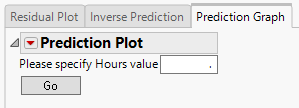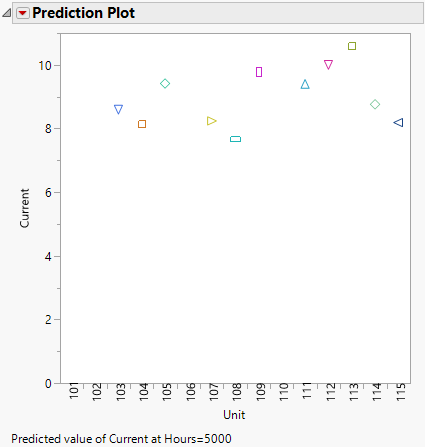Publication date: 08/13/2020

# Prediction Graph

Use the Prediction Graph tab to predict the Y variable for a specified Time value.

Figure 7.15 Prediction Plot TabFor the GaAs Laser example, no data was collected after 4000 hours. If you want to predict the percent increase in operating current after 5000 hours, enter 5000 and click Go. A plot is produced showing the estimated percent decrease after 5000 hours for all the units.

Figure 7.16 Prediction PlotThe Prediction Plot red triangle menu contains the following options:

Save Predictions

Saves the predicted Y values to a data table. When one of the Longitudinal Prediction Interval options is enabled, the table also includes the intervals.

Longitudinal Prediction Interval

Shows or hides confidence or prediction intervals for the estimated Y on the Prediction Plot. When intervals are enabled, the intervals are also included in the data table that is created when using the Save Predictions option.

Longitudinal Prediction Time

Specifies the time value for which you want to predict the Y.

Longitudinal Prediction Alpha

Specifies the alpha level used for the intervals.